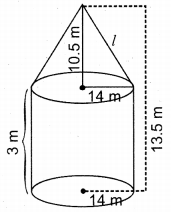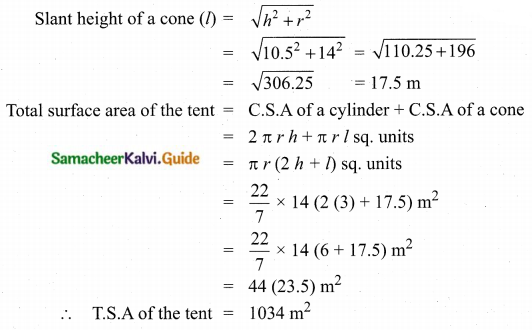Students can download Maths Chapter 7 Mensuration Additional Questions and Answers, Notes, Samacheer Kalvi 10th Maths Guide Pdf helps you to revise the complete Tamilnadu State Board New Syllabus, helps students complete homework assignments and to score high marks in board exams.

## Tamilnadu Samacheer Kalvi 10th Maths Solutions Chapter 7 Mensuration Additional Questions

I. Multiple Choice Questions:

Question 1.
The curved surface area of a right circular cylinder of radius 1 cm and height 1 cm is equal to ______
(1) π cm2
(2) 2π cm2
(3) 3π cm2
(4) 2 cm2
(2) 2π cm2
Hint:
C.S.A of a cylinder = 2πrh sq. units = 2 × π × 1 × 1 cm2 = 2π cm2

Question 2.
The total surface area of a solid right circular cylinder whose radius is half of its height h is equal to ______ sq. units.
(1) $$\frac{3}{2} \pi h$$
(2) $$\frac{2}{3} \pi h^{2}$$
(3) $$\frac{3}{2} \pi h^{2}$$
(4) $$\frac{2}{3} \pi h$$
(3) $$\frac{3}{2} \pi h^{2}$$
Hint:
T.S.A = 2πr(h + r)
[radius is half of the height]
= $$2 \pi \times \frac{h}{2}\left(\frac{h}{2}+h\right)$$
= $$=\pi h\left(\frac{3 h}{2}\right)=\frac{3}{2} \pi h^{2}$$ sq. unitsQuestion 3.
Base area of a right circular cylinder is 80 cm2. If its height is 5 cm, then the volume is equal to _______
(1) 400 cm3
(2) 16 cm3
(3) 200 cm3
(4) $$\frac{400}{3}$$ cm3
(1) 400 cm3
Hint:
Volume of a cylinder = πr2h cu. units
[Base area (πr2) = 80 cm2 = 80 × 5 cm3 = 400 cm3

Question 4.
If the total surface area of a solid right circular cylinder is 200π cm2 and its radius is 5 cm, then the sum of its height and radius is ______
(1) 20 cm
(2) 25 cm
(3) 30 cm
(4) 15 cm
(1) 20 cm
Hint:
T.S.A of a cylinder = 200π cm2
2πr (h + r) = 200π
2 × 5 (h + r) = 200
(h + r) = 20 cmQuestion 5.
The curved surface area of a right circular cylinder whose radius is a units and height is b units, is equal to ______
(1) πa2b sq.cm
(2) 2πab sq.cm
(3) 2π sq.cm
(4) 2 sq.cm
(2) 2πab sq.cm .
Hint:
C.S.A. of a cylinder = 2πrh sq. units = 2 × π × a × b sq. cm = 2πab sq. cm

Question 6.
Radius and height of a right circular cone and that of a right circular cylinder are respectively, equal. If the volume of the cylinder is 120 cm3, then the volume of the cone is equal to _______
(1) 1200 cm3
(2) 360 cm3
(3) 40 cm3
(4) 90 cm3
(3) 40 cm3
Hint:
Volume of the cone = $$\frac{1}{3}$$ × volume of the cylinder
= $$\frac{1}{3}$$ × 120 cm3
= 40 cm3Question 7.
If the diameter and height of a right circular cone are 12 cm and 8 cm respectively, then the slant height is
(1) 10 cm
(2) 20 cm
(3) 30 cm
(4) 96 cm
(1) 10 cm
Hint:
Slant height of a cone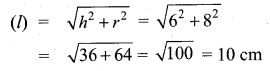Question 8.
If the circumference at the base of a right circular cone and the slant height are 120π cm and 10 cm respectively, then the curved surface area of the cone is equal to ______
(1) 1200π cm2
(2) 600π cm2
(3) 300π cm2
(4) 600 cm2
(2) 600π cm2
Hint:
Circumference (2πr) = 120π cm
Slant height (l) = 10 cm;
Curved surface area of a cone = πrl sq. units
= $$\frac{120 \pi}{2}$$ × 10 cm2 = 600π cm2Question 9.
If the volume and the base area of a right circular cone are 48π cm and 12π cm respectively, then the height of the cone is equal to ______
(1) 6 cm
(2) 8 cm
(3) 10 cm
(4) 12 cm
(4) 12 cm
Hint:
Volume of a cone = 48π cm3
[Base area (πr2) = 12π]
$$\frac{1}{3}$$ πr2h = 48π
$$\frac{1}{3}$$ × 12π × h = 48π
[Substitute πr2 = 12π]
h = $$\frac{48}{4}$$ = 12 cm

Question 10.
If the height and the base area of a right circular cone are 5 cm and 48 sq.cm respectively, then the volume of the cone is equal to _______
(1) 240 cm3
(2) 120 cm3
(3) 80 cm3
(4) 480 cm3
(3) 80 cm3
Hint:
Volume of a cone (V) = $$\frac{1}{3}$$ πr2h sq. units
Base area (πr2) = 48 sq. cm
V = $$\frac{1}{3}$$ × 48 × 5 = 80 cm3Question 11.
The ratios of the respective heights and the respective radii of two cylinders are 1 : 2 and 2 : 1 respectively. Then their respective volumes are in the ratio _______
(1) 4 : 1
(2) 1 : 4
(3) 2 : 1
(4) 1 : 2
(3) 2 : 1
Hint:
h1 : h2 = 1 : 2
r1 : r2 = 2 : 1
Ratio of their volumes
= $$\frac{1}{3} \pi r_{1}^{2} h_{1}: \frac{1}{3} \pi r_{2}^{2} h_{2}$$
= 22 × 1 : 12 × 2 = 4 : 2 = 2 : 1

Question 12.
If the radius of a sphere is 2 cm, then the curved surface area of the sphere is equal to ________
(1) 8π cm2
(2) 16 cm2
(3) 12π cm2
(4) 16π cm2
(4) 16π cm2
Hint:
C.S.A of a sphere = 4πr2 sq. units
= 4 × π × 22 cm2
= 16π cm2Question 13.
The total surface area of a solid hemisphere of diameter 2 cm is equal to _______
(1) 12 cm2
(2) 12π cm2
(3) 4π cm2
(4) 3π cm2
(4) 3π cm2
Hint:
Radius of a hemisphere = $$\frac{2}{2}$$ = 1 cm
Total surface area of a hemisphere = 3πr2 sq. units = 3 × π × 12 cm2 = 3π cm2

Question 14.
If the volume of a sphere is $$\frac{9}{16} \pi$$ cu.cm, then its radius is ________
(1) $$\frac{4}{3}$$ cm
(2) $$\frac{3}{4}$$ cm
(3) $$\frac{3}{2}$$ cm
(4) $$\frac{2}{3}$$ cm
(2) $$\frac{3}{4}$$ cm
Hint:
Volume of the sphere = $$\frac{9}{16} \pi$$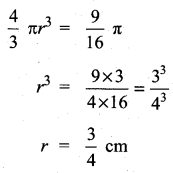Question 15.
The surface areas of two spheres are in the ratio of 9 : 25. Then their volumes are in the ratio _______
(1) 81 : 625
(2) 729 : 15625
(3) 27 : 75
(4) 27 : 125
(4) 27 : 125
Hint:
Ratio of their surface area = 9 : 25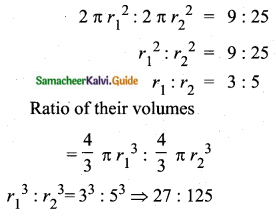Question 16.
The total surface area of a solid hemisphere whose radius is a units, is equal to ________
(1) 2πa2 sq. units
(2) 3πa2 sq. units
(3) 3πa sq. units
(4) 3a2 sq. units
(2) 3πa2 sq. units
Hint:
T.S.A. of a solid hemisphere = 3πr2 sq. units
= 3 × π × a × a sq.units
= 3πa2 sq. unitsQuestion 17.
If the surface area of a sphere is 100π cm2, then its radius is equal to ______
(1) 25 cm
(2) 100 cm
(3) 5 cm
(4) 10 cm
(3) 5 cm
Hint:
Surface area of a sphere = 100π cm2
4πr2 = 100π
r2 = 25
r = √25 = 5 cm

Question 18.
If the surface area of a sphere is 36π cm2, then the volume of the sphere is equal to _______
(1) 12π cm3
(2) 36π cm3
(3) 72π cm3
(4) 108π cm3
(2) 36π cm3
Hint:
Surface area of a sphere = 36π cm2
4πr2 = 36π
r2 = 9
r = 3 cm
Volume of a sphere = $$\frac{4}{3} \pi r^{3}$$ cu. units
= $$\frac{4}{3} \pi$$ × 3 × 3 × 3 cm3 = 36π cm3Question 19.
If the total surface area of a solid hemisphere is 12π cm2 then its curved surface area is equal to ______
(1) 6π cm2
(2) 24π cm2
(3) 36π cm2
(4) 8π cm2
(4) 8π cm2
Hint:
T.S.A of a hemisphere = 12π cm2
3πr2 = 12π
r2 = 4
r = 2
Curved surface area of a hemisphere = 2πr2 = 2 × π × 4 = 8π cm2

Question 20.
If the radius of a sphere is half of the radius of another sphere, then their respective volumes are in the ratio _____
(1) 1 : 8
(2) 2 : 1
(3) 1 : 2
(4) 8 : 1
(1) 1 : 8
Hint:
$$r_{1}=\frac{r_{2}}{2} \Rightarrow \frac{r_{1}}{r_{2}}=\frac{1}{2} \Rightarrow r_{1}: r_{2}=1: 2$$

Question 1.
Curved surface area and circumference at the base of a solid right circular cylinder are 4400 sq.cm and 110 cm respectively. Find its height and diameter.
Given, Circumference of the base of a cylinder = 110 cm
2πr = 110 ……. (1)
Curved surface area = 4400 cm2
2πrh = 4400 cm2 ……. (2)
From (1) & (2), $$\frac{(2)}{(1)} \Rightarrow \frac{2 \pi r h}{2 \pi r}=\frac{4400}{110}=40 \mathrm{cm}$$
Height of the cylinder (h) = 40 cm
From (1), 2πr = 110
2 × $$\frac{22}{7}$$ × r = 110
r = $$\frac{35}{2}$$
We know that, diameter (d) = 2 × radius
d = 2 × $$\frac{35}{2}$$ = 35 cm
Diameter of the Circular cylinder = 35 cmQuestion 2.
A mansion has 12 right cylindrical pillars each having radius 50 cm and height 3.5 m. Find the cost of painting the lateral surface of the pillars at ₹ 20 per square metre.
Given, Radius of a cylinder (r) = 50 cm = 0.5 m
Height of a cylinder (h) = 3.5 m
Curved surface area of a pillar = 2πrh sq. units
Curved surface area of 12 pillars = 12 × 2πrh
= 12 × 2 × $$\frac{22}{7}$$ × 0.5 × 3.5 m2
= 132 sq. m.
Cost for painting the lateral surface of pillars per metre = ₹ 20
Cost of painting = 132 × ₹ 20 = ₹ 2640

Question 3.
The total surface area of a solid right circular cylinder is 231 cm2. Its curved surface area is two thirds of the total surface area. Find the radius and height of the cylinder.
Given, Total surface area of a cylinder (T.S. A) = 231 sq.cm
Curved surface area = $$\frac{2}{3}$$ × T.S.A = $$\frac{2}{3}$$ × 231 = 154 cm2
2πrh = 154 cm2 …… (1)
Total surface area = 231 cm2
2πr (h + r) = 231
2πrh + 2πr2= 231
154 + 2πr2 = 231 [from (1)]
2πr2 = 231 – 154 = 77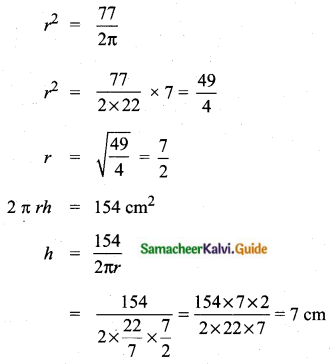Radius of the cylinder = 3.5 cm
Height of the cylinder = 7 cm

Question 4.
The total surface area of a solid right circular cylinder is 1540 cm2. If the height is four times the radius of the base, then find the height of the cylinder.
Given, Let the radius of the cylinder be ‘r’
Height of a cylinder (h) = 4r (by given condition)
Total surface area = 1540 cm2
2πr(h + r) = 1540 cm2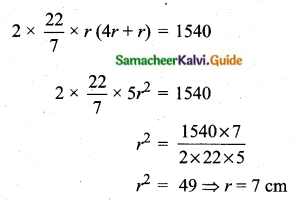Height of the cylinder = 4r = 4 × 7 = 28 cm

Question 5.
If the vertical angle and the radius of a right circular cone are 60° and 15 cm respectively, then find its height and slant height.
Given, In the figure, OAB is a cone and OC ⊥ AB
∠AOC = $$\frac{60^{\circ}}{2}$$ = 30°
In the right ∆OAC, tan 30° = $$\frac{\mathrm{AC}}{\mathrm{OC}}$$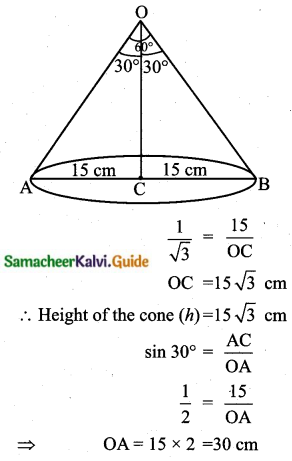Slant Height of the cone (l) = 15 × 2 = 30 cm

Question 6.
The central angle and radius of a sector of a circular disc are 180° and 21 cm respectively. If the edges of the sector are joined together to make a hollow cone, then find the radius of the cone.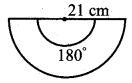Given, Radius of a sector (r) = 21 cm
The angle of the sector (θ) = 180°
Let “R” be the radius of the cone.
Circumference of the base of a cone = Arc length of the sector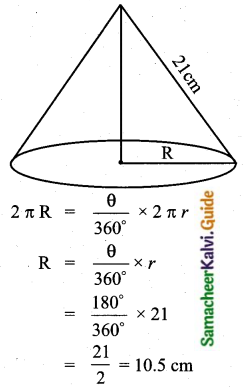Radius of the cone (R) = 10.5 cm

Question 7.
If the curved surface area of a solid hemisphere is 2772 sq.cm, then find its total surface area.
Given, the Curved surface area of a solid hemisphere = 2772 cm2
2πr2 = 2772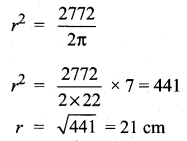Total surface area = 3πr2 sq. units
= 3 × $$\frac{22}{7}$$ × 21 × 21
= 4158 sq.cm
Aliter:
C.S.A of a hemisphere = 2772 cm2
2πr2 = 2772 cm2
πr2 = $$\frac{2772}{2}$$ = 1386 cm
T.S.A of a hemisphere = 3πr2 sq.units = 3 × 1386 cm2 = 4158 cm2

Question 8.
An inner curved surface area of a hemispherical dome of a building needs to be painted. If the circumference of the base is 17.6 m, find the cost of painting it at the rate of ₹ 5 per sq. m.
Given, Circumference of the dome = 17.6 m
2πr = 17.6
$$r=\frac{17.6 \times 7}{2 \times 22}=\frac{8.8 \times 7}{22}=2.8 \mathrm{m}$$
Curved surface area of the dome = 2πr2 sq. units
= 2 × $$\frac{22}{7}$$ × 2.8 × 2.8 m2
= 49.28 m2
Cost of painting for one sq.metre = ₹ 5
Cost of painting the curved surface = 49.28 × ₹ 5 = ₹ 246.40

Question 9.
Volume of a solid cylinder is 62.37 cu.cm. Find the radius if its height is 4.5 cm.
Given, Height of a cylinder (h) = 4.5 cm
Volume of a solid cylinder = 62.37 cu. cm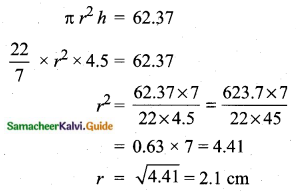Radius of a cylinder (r) = 2.1 cm

Question 10.
A rectangular sheet of metal foil with dimension 66 cm × 12 cm is rolled to form a cylinder of height 12 cm. Find the volume of the cylinder.
After rolling the rectangular sheet into a cylinder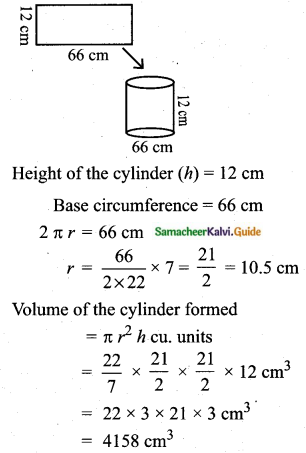Volume of the cylinder = 4158 cm3

Question 11.
The circumference of the base of a 12 m high wooden solid cone is 44 m. Find the volume.
Given, Height of the wooden solid cone (h) = 12 m
Circumference of the base = 44 m
2πr = 44
r = $$\frac{44 \times 7}{2 \times 22}$$ = 7 m
Volume of the wooden solid = $$\frac{1}{3} \pi r^{2} h$$ cu. units
= $$\frac{1}{3} \times \frac{22}{7} \times 7 \times 7 \times 12 \mathrm{m}^{3}$$
= 88 × 7
= 616 m3
Volume of the solid = 616 m3

Question 12.
Find the volume of the largest right circular cone that can be cut out of a cube whose edge is 14 cm.
Given, Edge of the cube = 14 cm
The largest circular cone is cut out from the cube.
Radius of the cone (r) = $$\frac{14}{2}$$ = 7 cm
Height of the cone (h) = 14 cm
Volume of a cone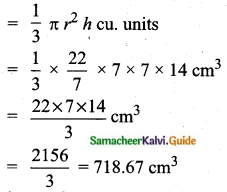Volume of a cone = 718.67 cm3

Question 13.
The thickness of a hemispherical bowl is 0.25 cm. The inner radius of the bowl is 5 cm. Find the outer curved surface area of the bowl. (Take π = $$\frac{22}{7}$$)
Let r, R and w be the inner and outer radii and thickness of the hemispherical bowl respectively.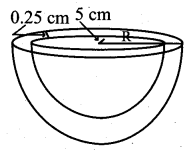Given that r = 5 cm, w = 0.25 cm
R = r + w = 5 + 0.25 = 5.25 cm
Now, outer surface area of the bowl = 2πR2
= 2 × $$\frac{22}{7}$$ × 5.25 × 5.25
= 173.25 sq. cm
Thus, the outer surface area of the bowl = 173.25 sq. cm

Question 14.
Volume of a hollow sphere is $$\frac{11352}{7}$$ cm3. If the outer radius is 8 cm, find the inner radius of the sphere. (Take π = $$\frac{22}{7}$$)
Let R and r be the outer and inner radii of the hollow sphere respectively.
Let V be the volume of the hollow sphere.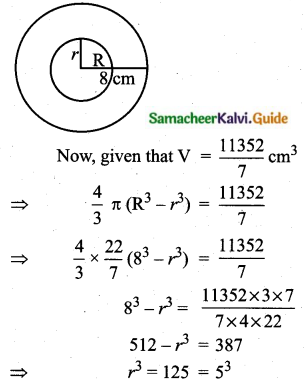Hence, the inner radius r = 5 cm

Question 15.
How many litres of water will a hemispherical tank whose diameter is 4.2 m?
Radius of the tank = $$\frac{4.2}{2}$$ = 2.1 m
Volume of the hemisphere
= $$\frac{2}{3} \pi r^{3}$$ cu.units
= $$\frac{2}{3} \times \frac{22}{7} \times 2.1 \times 2.1 \times 2.1 \mathrm{m}^{3}$$
= 19.404 m3
= 19.404 x 1000 lit
= 19,404 litres

Question 1.
A vessel is in the form of a hollow hemisphere mounted by a hollow cylinder. The diameter of the hemisphere is 14 cm and the total height of the vessel is 13 cm. Find the inner surface area of the vessel.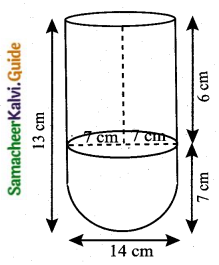For cylindrical part:
Height (h) = 6 cm
Curved surface area = 2πrh = 2 × $$\frac{22}{7}$$ × 7 × 6 cm2 = 264 cm2
For hemispherical part:
Surface area (h) = 2πr2
= 2 × $$\frac{22}{7}$$ × 7 × 7 cm2
= 308 cm2
Total surface area = (264 + 308) = 572 cm2

Question 2.
A medicine capsule is in the shape of a cylinder with two hemispheres stuck to each of its ends. The length of the entire capsule is 14 mm and the diameter of the capsule is 5 mm. Find its surface area.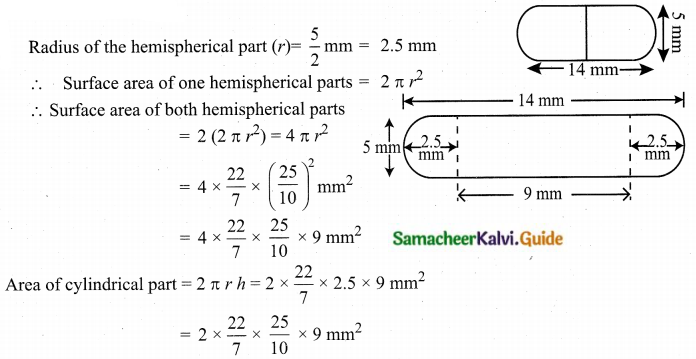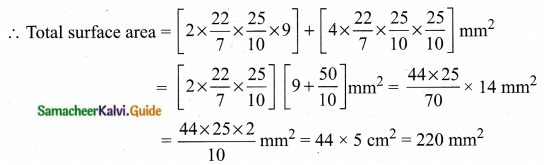Question 3.
From a solid cylinder whose height is 2.4 cm and diameter 1.4 cm, a conical cavity of the same height and same diameter is hollowed out. Find the total surface area of the remaining solid to the nearest cm2.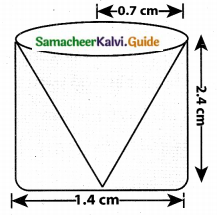For cylinderical part:
Height (h) = 2.4 cm
Diameter (d) = 1.4 cm
Total surface area of the cylindrical part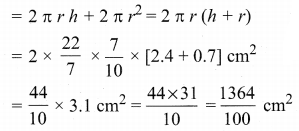For conical part:
Base area (r) = 0.7 cm
Height (h) = 2.4 cm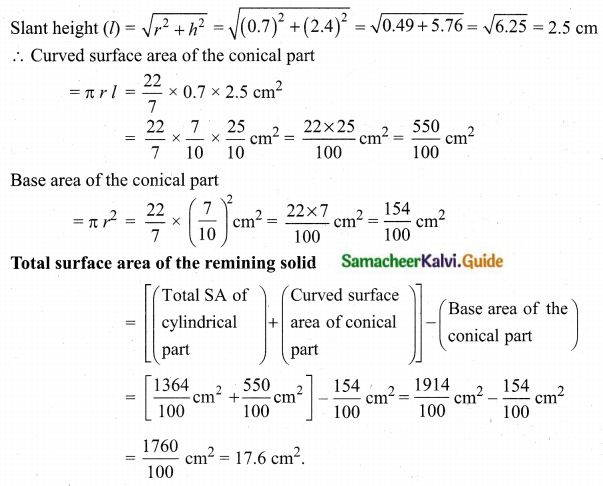Question 4.
A 20 m deep well with diameter 7 m is dug and the earth from digging is evenly spread out to form a platform 22 m by 14 m. Find the height of the platform.
Diameter of the cylindrical well = 7 m
Radius of the cylinder (r) = $$\frac{7}{2}$$ m
Depth of the well (h) = 20 m
Volume = πr2h
= $$\frac{22}{7} \times \frac{7}{2} \times \frac{7}{2} \times 20 \mathrm{m}^{3}$$
= 22 × 7 × 5 m3
Volume of the earth taken out = 22 × 7 × 5 m3
Now this earth is spread out to form a cuboidal platform having
Length (l) = 22 m
Let ‘h’ be the height of the platform.
Volume of the platform = 22 × 14 × h m3
Volume of the platform = Volume of the earth taken out
22 × 14 × h = 22 × 7 × 5
$$h=\frac{22 \times 7 \times 5}{22 \times 14}=\frac{5}{2} \mathrm{m}=2.5 \mathrm{m}$$
Thus, the required height of the platform is 2.5 m.

Question 5.
The perimeters of the ends of the frustum of a cone are 207.24 cm and 169.56 cm. If the height of the frustum is 8 cm, find the whole surface area of the frustum. [Use π = 3.14]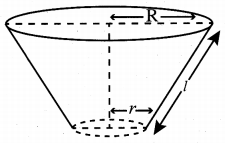Let the radii of circular ends are R and r [R > r]
Perimeter of circular ends are 207.24 cm and 169.56 cm
2πR = 207.24 cm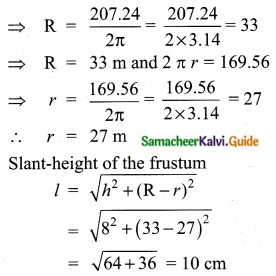The whole surface area of the frustum = π [(R2 + r2) + (R + r) l]
Required whole surface area of the frustum
= 3.14 [332 + 272 + (33 + 27) × 10] cm2
= 3.14 [1089 + 729 + 600] cm2
= 3.14  cm2
= 7592.52 cm2

Question 6.
A cuboid-shaped slab of iron whose dimensions are 55 cm × 40 cm × 15 cm is melted and recast into a pipe. The outer diameter and thickness of the pipe are 8 cm and 1 cm respectively. Find the length of the pipe. (Take π = $$\frac {22}{7}$$)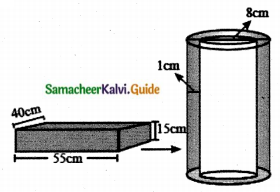Let h1 be the length of the pipe
Let R and r be the outer and inner radii of the pipe respectively.
Iron slab:
Volume = lbh = 55 × 40 × 15 cm3
Iron pipe:
Outer diameter, 2R = 8 cm
Outer radius, R = 4 cm
Thickness, w = 1 cm
Inner radius, r = R – w = 4 – 1 = 3 cm
Now, the volume of the iron pipe = Volume of the iron slab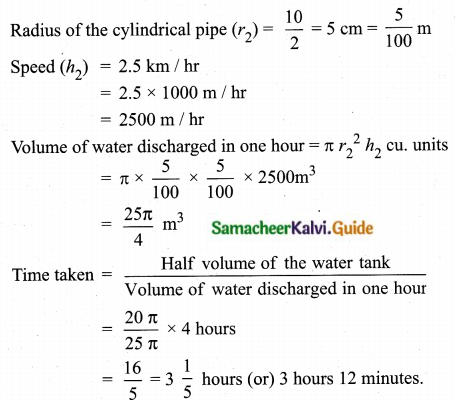Time is taken by the pipe to empty half of the tank = 3 hours 12 minutes.

Question 7.
The perimeter of the ends of a frustum of a cone are 44 cm and 8.4π cm. If the depth is 14 cm., then find its volume.
Given let the radius of the top of the frustum be “R” and the radius of the bottom of the frustum be “r”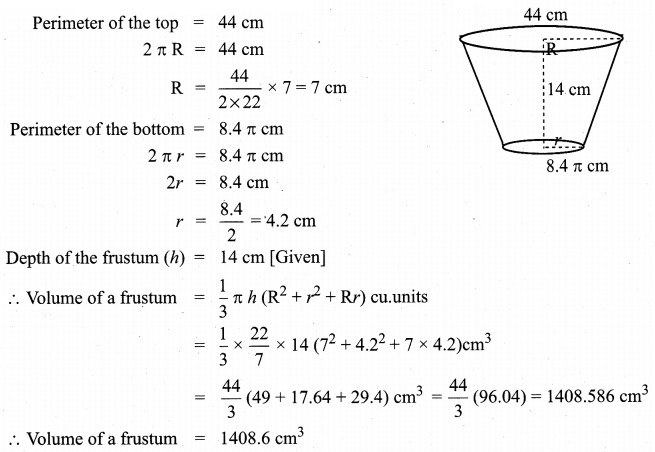Question 8.
A tent is in the shape of a right circular cylinder surmounted by a cone. The total height and the diameter of the base are 13.5 m and 28 m. If the height of the cylindrical portion is 3 m, find the total surface area of the tent.